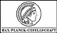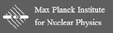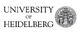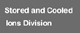Superordinated navigation: MPIK Homepage  |  Home  |  Deutsch  |  Sitemap  |  Search  |  Contact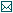Contact

Tel.: +49 6221 516-851
Fax: +49 6221 516-852
Max Planck Institute for Nuclear Physics
P.O. Box 10 39 80
69029 Heidelberg
Max Planck Institute for Nuclear Physics
Saupfercheckweg 1
Building: Gentner lab,
room 134
69117 Heidelberg

# THe-TRAP Project

## Simulations

To understand the experiment and the deviation from the ideal mathematical description better, various simulations are performed by the group. For this there are several tools available such as Matlab, Tosca Vector Fields Opera, SimIon and Comsol Multiphysics. In the following, a brief overview of already examined areas is given.

### Electrostatics

The hyperbolic electrodes of the Penning traps are different from the ideal shape due to manufacturing tolerances, the ability to transport ions through the end cap and the simple inability to make them infinitely large. In order to understand these (and other) effects on the fields in the trap in more detail, simulations were performed with Comsol Multiphysics. Comsol Multiphysics is a simulation tool that uses the finite element method for the simulation of different physical scenarios. For calculating the solution, a grid is placed on the defined geometry and the differential equation for each of the underlying problem is solved at the grid points. An advantage of Comsol Multiphysics is that it can use existing symmetries. Thus in most cases, the electrostatic simulation of THe-Trap can be considered as a rotationally symmetric problem. An example of the THe-Trap trap-geometry with a grid can be seen in Figure 7.1.Fig. 7.1: THe-Trap electrode geometry implemented in Comsol Multiphysics, using rotational symmetry. The full trap is obtained by rotating the cross-section around the symmetry axis (red dotted line). For the electric field insubstantial parts are removed. The grid for the calculation of the electric field is generated only outside of the electrode, since the interior of the electrodes is field-free.

^ to the top

The simulated electric potential created with -90V at ring and -45V applied to the correction electrodes is shown in Figure 7.2. These are typical values for the experiment, because then the axial frequency of T+, 3He+ and 12C4+ is close to the 4 MHz chosen for the axial detection system.Fig. 7.2: On the ring -90V and on the correction electrodes -45V are applied. These are typical values for the experiment. The color gradient shows the simulated profile of the electric potential. The legend is here multiplied with -1.